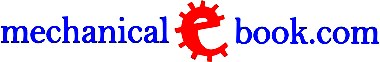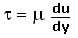Custom Search

 Fluid Mechanics and MachinesFluid : A fluid is defined as a substance that contiguously deforms when subjected to a shear stress regardless of how small the shear stress may be. It may be a gas, liquid or fluidized solid powder and is considered to consist of finite particles each much larger than a molecule but infinitesimal in size compared to total volume of fluid.

Ideal Fluid : It is frictionless and incompressible. In other words, a fluid which has no resistance to shear stress is known as an ideal fluid. A Newtonian fluid is a fluid whose stress versus rate of strain curve is linear and passes through the origin.

Discharge : It is a volume of water which flows through a section of a channel or a pipe per second.

Pascal's Law : It states that 'when a pressure is applied to a fluid which is enclosed in a container is transmitted to every part of fluid and to the surfaces of its container.

Path Line : The path which is followed by a fluid particle in motion. In other words, Path Line is the trajectory that individual fluid particles follow.

Stream Line : It is a family of curves that are instantaneously tangent to the velocity vector of the flow.

Streak Line : It is an instantaneous position of all fluid particles which have passed through a given point.

Potential Line or Equipotential Line : The line which joins the points of equal potential on adjacent flow lines. They provide a quantitative way to view the electric potential in two dimensions.

Flow Net : It is a pattern which is obtained by the intersection of stream lines and potential lines. It is often used for solving groundwater flow problems where the geometry makes analytical solutions impractical.

Uniform Flow : The type of flow in which the velocities of liquid particles at all sections of the pipe or channel are equal, is called Uniform Flow.

Non-uniform Flow : The type of flow in which velocities of liquid particles at all sections of the pipe or channel are not equal, is called Non-uniform Flow.

Steady Flow : The type of flow in which the quantity of liquid flowing per second is constant, is called Steady Flow.

Unsteady Flow : The type of flow in which the quantity of liquid flowing per second is not constant, is called Unsteady Flow.

Laminar Flow : The type of flow in which paths of individual particles do not cross each other, is called Laminar Flow. Reynold's number is less than 2000 for laminar flow.

Turbulent Flow : The type of flow in which the paths of individual particles cross each other and particles do not have definite paths, is called Turbulent Flow. Reynold's number is more than 4000 for turbulent flow.

One dimensional Flow : The type of flow whose stream lines may be represented by straight line, is called One dimensional Flow.

Two dimensional Flow : The type of liquid flow whose stream lines may be represented by a curve, is called Two dimensional Flow.

Vortex Flow : Rotation of a fluid particle about a given axis is defined as the average angular velocity of two infinitesimal line elements in the particle which are to the given axis and at right angles to each other. The flow is called Vortex Flow when the fluid particles within a region have rotation about any axis. It is also called Rotational Flow.

Viscosity : It is a measure of the resistance of a fluid which is being deformed by either shear stress or extensional stress. In other words, it is the resistance of a liquid to flow, or its thickness. It describes a fluid's internal resistance to flow and may be thought of as a measure of fluid friction.

Kinematic Viscosity : The ratio of absolute or dynamic viscosity and the density. In other words, the dynamic viscosity which is divided by the density for a Newtonian fluid. It is partially independent of pressure and depends upon temperature only.

Newton's Law of Viscosity : Shear stress,For straight, parallel and uniform flow, the shear stress, t, between layers is proportional to the velocity gradient (m), du/dy, in the direction perpendicular to the layers.